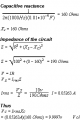Current in capacitor RC

ironasona

Joined Nov 12, 2020
53
Hi , i was just wondering how would i find the current through a capacitor (Series RC circuit)

I found a questions online which asks to find the voltage and the current through a capacitor at 1kHz and 10Khz

Capacitor = 0.01uF
Resistor = 100 Ohms
Voltage Source = 10vModerator edit: post restored to it’s original content.
Please do not edit your post following a long discussion. It makes fools of all of us.

Attachments

Last edited by a moderator:

Externet

Joined Nov 29, 2005
1,732
The current is the voltage (100V) divided by the impedance.

ironasona

Joined Nov 12, 2020
53
The current is the voltage (100V) divided by the impedance.
so voltage across capacitor = 0.05263A?

Externet

Joined Nov 29, 2005
1,732
I typed or misread the voltage wrong. You specified 10, I wrongly put 100 above•ironasona

MrChips

Joined Oct 2, 2009
25,004
so voltage across capacitor = 0.05263A?
No. Voltage cannot have units of A.

•djsfantasi

ironasona

Joined Nov 12, 2020
53
I typed or misread the voltage wrong. You specified 10, I wrongly put 100 aboveso what would be the current through a capacitor ? just 0.05263?, kinda lost sorry

MrChips

Joined Oct 2, 2009
25,004
Since R and C are in series the current in C must be the same as the current in R.
Therefore, calculate the current in R.

What is the total impedance of the circuit?

MrChips

Joined Oct 2, 2009
25,004
Ok
Current Irms = Vrms / Ztotal

Voltage Source = 10V
We don't know what this means.
I would read this to mean Voltage Source = 10VDC.

ironasona

Joined Nov 12, 2020
53
yes, so 10vdc/190ohms = 0.05263 ?
is it the same thing happen if the circuit is AC ?

MrChips

Joined Oct 2, 2009
25,004
is it the same thing happen if the circuit is AC ?
The circuit is not defined as AC or DC.
You need to define the excitation voltage as a function of time.

Edit: please do not edit your post following a long discussion on the matter. It makes fools of all of us.

btw,
1μF = 0.000001F = 1 x 10^-6 F

MrAl

Joined Jun 17, 2014
8,607
Hi , i was just wondering how would i find the current through a capacitor (Series RC circuit)

I found a questions online which asks to find the voltage and the current through a capacitor at 1kHz and 10Khz

Capacitor = 0.01uF
Resistor = 100 Ohms
Voltage Source = 10v

View attachment 242906
Moderator edit: post restored to it’s original content.
Please do not edit your post following a long discussion. It makes fools of all of us.
By that post i would say this is an AC analysis problem.
What i dont get though it why there are three different cap values: 0.01uf, 1e-4 F, and 1e-6 F.
With 1e-6F i get a similar result but mine comes out to 0.0532018 to that many digits. Units in correlated units volts to amps (peak, peak, or RMS, RMS, etc.). So my result is slightly different using 1e-6 Farads.
I also followed suit with f=1000Hz and R=100 Ohms.